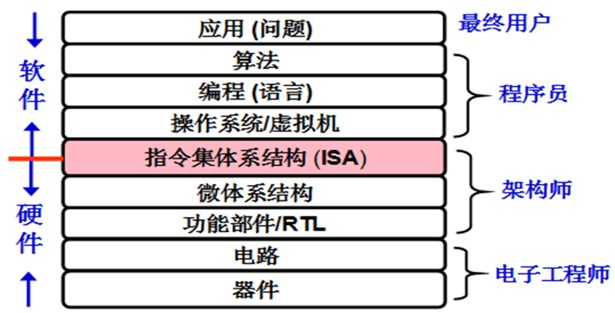## 为什么要学习计算机系统基础

### 一知半解

#### 数组求和

``````int sum(int a[ ], unsigned len) {
int i, sum = 0;
for (i = 0; i <= len-1; i++)
sum += a[i];
return sum;
}
``````

`len = 0`时, 执行`sum`函数的for循环时会发生`Access Violation`, 即"访问违例"异常. 但是, 当参数`len`说明为`int`型时, `sum`函数能正确执行, 为什么?

#### 整数的平方

`x``y``int`型, 当`x = 65535`时, 则`y = x*x = -131071`. 为什么?

#### 多重定义符号

``````/*---main.c---*/
#include <stdio.h>
int d=100;
int x=200;
void p1(void);
int main() {
p1();
printf("d=%d,x=%d\n",d,x);
return 0;
}

/*---p1.c---*/
double d;
void p1() {
d=1.0;
}
``````

#### 奇怪的函数返回值

``````double fun(int i) {
volatile double d = {3.14};
volatile long int a;
a[i] = 1073741824; /* Possibly out of bounds */
return d;
}
``````

`fun`函数的源码来看, 每次返回的值应该都是`3.14`, 可是执行`fun`函数后发现其结果是:

• `fun(0)``fun(1)``3.14`
• `fun(2)``3.1399998664856`
• `fun(3)``2.00000061035156`
• `fun(4)``3.14`并会发生`访问违例` 这是为什么?

#### 时间复杂度和功能都相同的程序

``````void copyij(int src, int dst) {
int i,j;
for (i = 0; i < 2048; i++)
for (j = 0; j < 2048; j++)
dst[i][j] = src[i][j];
}
void copyji(int src, int dst) {
int i,j;
for (j = 0; j < 2048; j++)
for (i = 0; i < 2048; i++)
dst[i][j] = src[i][j];
}
``````

#### 网友贴出的一道百度招聘题

``````#include <stdio.h>
int main() {
double a = 10;
printf("a = %d\n", a);
return 0;
}
``````

#### 整数除法

• 代码段一:
``````int a = 0x80000000;
int b = a / -1;
printf("%d\n", b);
``````
• 代码段二:
``````int a = 0x80000000;
int b = -1;
int c = a / b;
printf("%d\n", c);
``````
代码段一的运行结果为`-2147483648`; 而代码段二的运行结果为`Floating point exception`. 显然, 代码段二运行时被检测到了"溢出"异常. 看似同样功能的程序为什么结果完全不同?

### 站得高, 看得远• 能够对软, 硬件功能进行合理划分
• 能够对系统不同层次进行抽象和封装
• 能够对系统的整体性能进行分析和调优
• 能够对系统各层面的错误进行调试和修正
• 能够根据系统实现机理对用户程序进行准确的性能评估和优化
• 能够根据不同的应用要求合理构建系统框架等

• 对计算机系统整机概念的认识
• 对计算机系统层次结构的深刻理解
• 对高级语言程序, ISA, OS, 编译器, 链接器等之间关系的深入掌握
• 对指令在硬件上执行过程的理解和认识
• 对构成计算机硬件的基本电路特性和设计方法等的基本了解等 从而能够更深刻地理解时空开销和权衡, 抽象和建模, 分而治之, 缓存和局部性, 吞吐率和时延, 并发和并行, 远程过程调用(RPC), 权限和保护等重要的核心概念, 掌握现代计算机系统中最核心的技术和实现方法.

`计算机系统基础`课程的主要教学目标是培养学生的系统能力, 使其成为一个"高效"程序员, 在程序调试, 性能提升, 程序移植和健壮性等方面成为高手; 建立扎实的计算机系统概念, 为后续的OS, 编译, 体系结构等课程打下坚实基础.

### 实践是检验真理的唯一标准

• (theory, 理论计算机科学) 什么是计算?
• (system, 计算机系统) 什么是计算机?
• (application, 计算机应用) 我们能用计算机做什么?

##### 终极拷问

PhotoShop里面的滤镜功能繁多, 随便点点就能美化图片, 为什么还需要学习图像处理的基本原理?

##### 宋公语录

此页面修订于: 2023-05-13 13:14:10